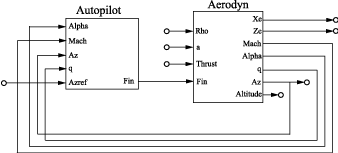# MIMO Feedback Loop

This example shows how to obtain the closed-loop response of a MIMO feedback loop in three different ways.

In this example, you obtain the response from `Azref` to `Az` of the MIMO feedback loop of the following block diagram.You can compute the closed-loop response using one of the following three approaches:

• Name-based interconnection with `connect`

• Name-based interconnection with `feedback`

• Index-based interconnection with `feedback`

You can use whichever of these approaches is most convenient for your application.

Load the plant `Aerodyn` and the controller `Autopilot` into the MATLAB® workspace. These models are stored in the datafile `MIMOfeedback.mat`.

`load('MIMOfeedback.mat')`

`Aerodyn` is a 4-input, 7-output state-space (`ss`) model. `Autopilot` is a 5-input, 1-output `ss` model. The inputs and outputs of both models names appear as shown in the block diagram.

Compute the closed-loop response from `Azref` to `Az` using `connect`.

`T1 = connect(Autopilot,Aerodyn,'Azref','Az');`
```Warning: The following block inputs are not used: Rho,a,Thrust. ```
```Warning: The following block outputs are not used: Xe,Ze,Altitude. ```

The `connect` function combines the models by joining the inputs and outputs that have matching names. The last two arguments to `connect` specify the input and output signals of the resulting model. Therefore, `T1` is a state-space model with input `Azref` and output `Az`. The `connect` function ignores the other inputs and outputs in `Autopilot` and `Aerodyn`.

Compute the closed-loop response from `Azref` to `Az` using name-based interconnection with the `feedback` command. Use the model input and output names to specify the interconnections between `Aerodyn` and `Autopilot`.

When you use the `feedback` function, think of the closed-loop system as a feedback interconnection between an open-loop plant-controller combination `L` and a diagonal unity-gain feedback element `K`. The following block diagram shows this interconnection.```L = series(Autopilot,Aerodyn,'Fin'); FeedbackChannels = {'Alpha','Mach','Az','q'}; K = ss(eye(4),'InputName',FeedbackChannels,... 'OutputName',FeedbackChannels); T2 = feedback(L,K,'name',+1);```

The closed-loop model `T2` represents the positive feedback interconnection of `L` and `K`. The `'name'` option causes `feedback` to connect `L` and `K` by matching their input and output names.

`T2` is a 5-input, 7-output state-space model. The closed-loop response from `Azref` to `Az` is `T2('Az','Azref')`.

Compute the closed-loop response from `Azref` to `Az` using `feedback`, using indices to specify the interconnections between `Aerodyn` and `Autopilot`.

```L = series(Autopilot,Aerodyn,1,4); K = ss(eye(4)); T3 = feedback(L,K,[1 2 3 4],[4 3 6 5],+1);```

The vectors `[1 2 3 4]` and `[4 3 6 5]` specify which inputs and outputs, respectively, complete the feedback interconnection. For example, `feedback` uses output 4 and input 1 of `L` to create the first feedback interconnection. The function uses output 3 and input 2 to create the second interconnection, and so on.

`T3` is a 5-input, 7-output state-space model. The closed-loop response from `Azref` to `Az` is `T3(6,5)`.

Compare the step response from `Azref` to `Az` to confirm that the three approaches yield the same results.

`step(T1,T2('Az','Azref'),T3(6,5),2)`